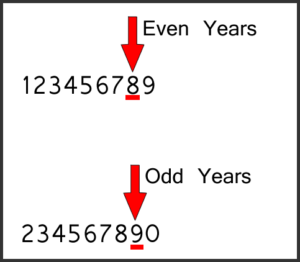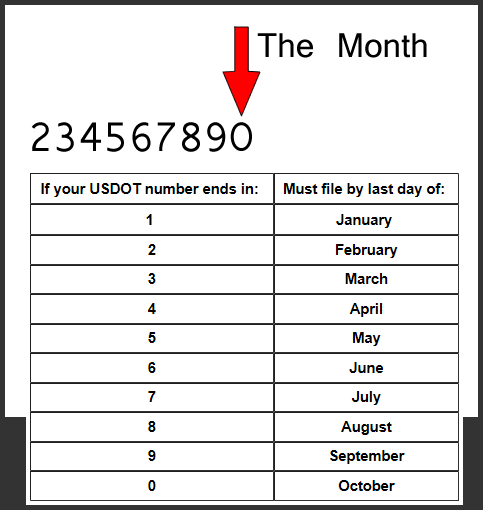# DOT Update Due Date Breakdown

If you have a US DOT number, then you probably already know it needs to be updated once every two years. This is called a biennial update.

But when exactly is your due date?? The FMCSA SAFER System tells you when you last renewed but doesn’t exactly post a “due date” anywhere! That’s because the due date is buried in the actual DOT number.

The last two digits of your DOT number hold the key.

## The Last Two Digits

Let’s say the last two digits of my DOT number are 12 – just as an example.

• The number 1 tells me which years the DOT update is due.
• The number 2 tells me what MONTH of that year the DOT Update is due.

### The Number One Spot

The number “1” spot in our example tells you if your biannual update is due in even years, or odd years. If the number in that space is Even then your biannual update is due in every even year and if the number in that space is Odd then your biannual update is due every odd year.### The Number Two Spot

Now that we know the year your updates are due, we need to know what month. The number “2” spot in our example explains. Check out the chart below.1 = January/2 = February/3 = March/4 = April/5 = May/6 = June/7 = July/8 = August/9 = September/0 = October

Figuring Your Due Date. Let’s get back to my DOT number that ends in 12 and figure out my Due Date. With a DOT ending in 12, I would be due in February of each odd year, 2013, 2015, 2017, 2019….

Now, pull out your DOT number and give it a once over. I bet it won’t take very long at all to figure out the Due Date.

## Real People

Call us here at 2290Tax.com if you need help figuring this out, or with any other trucking questions. There’s no magic to it, if you know your DOT number we can help you figure the right Due Date.

Written by Casey Jenkins

Posted on